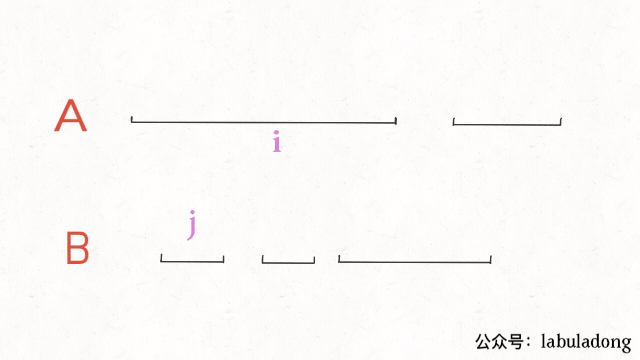## 总结

1、排序。常见的排序方法就是按照区间起点排序，或者先按照起点升序排序，若起点相同，则按照终点降序排序。当然，如果你非要按照终点排序，无非对称操作，本质都是一样的。

2、画图。就是说不要偷懒，勤动手，两个区间的相对位置到底有几种可能，不同的相对位置我们的代码应该怎么去处理。

## 区间覆盖``````int removeCoveredIntervals(int[][] intvs) {
// 按照起点升序排列，起点相同时终点降序排列
Arrays.sort(intvs, (a, b) -> {
if (a == b) {
return b - a;
}
return a - b;
});

// 记录合并区间的起点和终点
int left = intvs;
int right = intvs;

int res = 0;
for (int i = 1; i < intvs.length; i++) {
int[] intv = intvs[i];
// 情况一，找到覆盖区间
if (left <= intv && right >= intv) {
res++;
}
// 情况二，找到相交区间，合并
if (right >= intv && right <= intv) {
right = intv;
}
// 情况三，完全不相交，更新起点和终点
if (right < intv) {
left = intv;
right = intv;
}
}

return intvs.length - res;
}
``````

## 区间合并问题

``````# intervals 形如 [[1,3],[2,6]...]
def merge(intervals):
if not intervals: return []
# 按区间的 start 升序排列
intervals.sort(key=lambda intv: intv)
res = []
res.append(intervals)

for i in range(1, len(intervals)):
curr = intervals[i]
# res 中最后一个元素的引用
last = res[-1]
if curr <= last:
# 找到最大的 end
last = max(last, curr)
else:
# 处理下一个待合并区间
res.append(curr)
return res
``````

## 区间交集问题``````# A, B 形如 [[0,2],[5,10]...]
def intervalIntersection(A, B):
i, j = 0, 0 # 双指针
res = []
while i < len(A) and j < len(B):
a1, a2 = A[i], A[i]
b1, b2 = B[j], B[j]
# 两个区间存在交集
if b2 >= a1 and a2 >= b1:
# 计算出交集，加入 res
res.append([max(a1, b1), min(a2, b2)])
# 指针前进
if b2 < a2: j += 1
else:       i += 1
return res
``````